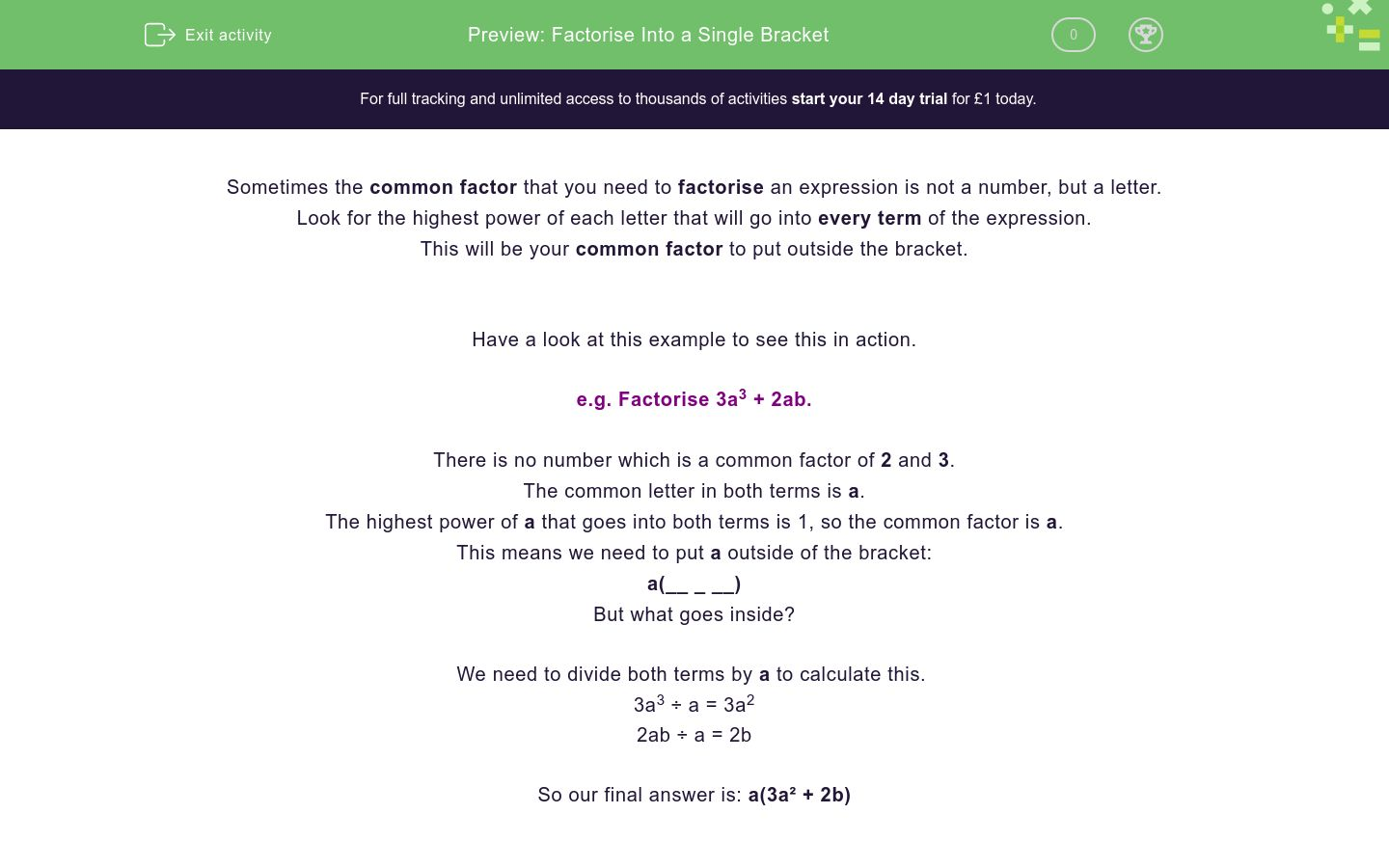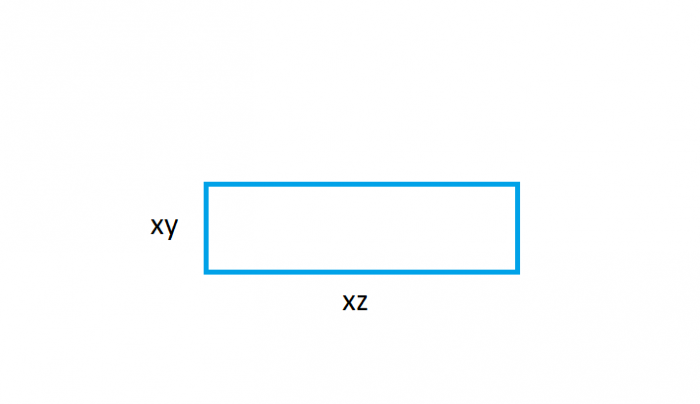# Factorise Into a Single Bracket

In this worksheet, students will factorise expressions into a single bracket using letters or numbers as common factors.Key stage:  KS 4

GCSE Subjects:   Maths

GCSE Boards:   Pearson Edexcel, OCR, Eduqas, AQA

Curriculum topic:   Algebra

Curriculum subtopic:   Notation, Vocabulary and Manipulation, Algebraic Expressions

Difficulty level:### QUESTION 1 of 10

Sometimes the common factor that you need to factorise an expression is not a number, but a letter.

Look for the highest power of each letter that will go into every term of the expression.

This will be your common factor to put outside the bracket.

Have a look at this example to see this in action.

e.g. Factorise 3a3 + 2ab.

There is no number which is a common factor of 2 and 3.

The common letter in both terms is a.

The highest power of a that goes into both terms is 1, so the common factor is a.

This means we need to put a outside of the bracket:

a(__ _ __)

But what goes inside?

We need to divide both terms by a to calculate this.

3a3 ÷ a = 3a2

2ab ÷ a = 2b

So our final answer is: a(3a² + 2b)

In this activity, we will factorise expressions into a single bracket using letters or numbers as common factors.

Match each expression below to its factorised form.

## Column B

p² + 9p
p(p + 9)
2p² - 9p
p(2p - 9)
2p³ + 9p²
p²(2p + 9)
2p² - 9p³
p²(2 - 9p)

True or false?

2x² + 5x = x(x + 5)

True

False

Jenny factorised an expression and got ab(4 + c) as her answer.

What was the question?

ab + 4abc

ab + 4c

4ab + abc

4ab - abc

Tick the expressions below which have been factorised correctly.

6a² - 7a = a(6a - 7)

6a - 7a²b = a(6 - 7b)

6a² + 7ab = a(6a + 7b)

6a² + 7a²b = a²(6 + 7ab)

What is the correct factorised form of 4x³y + 5xy?

xy(4x + 5)

xy(4x² + 5)

x(4x²y + 5y)

y(4x³ + 5x)

Match each expression below to its correct factorised form.

## Column B

3y³ - 5xy²
y²(2 + 3x)
2y² + 3xy²
xy(3y² - 5x)
3xy³ - 5x²y
y²(3y - 5x)
2x²y³ + 3xy²
xy²(2xy + 3)

Factorise:

5s³ - 7s²t²

s²(5s - 7t)

s²(5 - 7t²)

s²(5s - 7st²)

s²(5s - 7t²)

Tick the expressions which cannot be factorised.

2x² - xy

12x + 7y

x³ + 2y³

12xy + 5x²y

Which expression below correctly represents the perimeter of this rectangle?xy + z

2xy + z

x(y + z)

2x(y + z)

Factorise:

3x³y - 6x²y

xy(3x² - 6x)

3x²y(x - 2)

x²y(3x - 6)

3x²y(x - 6)

• Question 1

Match each expression below to its factorised form.

## Column B

p² + 9p
p(p + 9)
2p² - 9p
p(2p - 9)
2p³ + 9p²
p²(2p + 9)
2p² - 9p³
p²(2 - 9p)
EDDIE SAYS
Did you manage to find the common factors here? The final two questions are the trickiest. The common factor of 2p³ and 9p² is p² because it goes into both p³ and p². Therefore, we can put p² outside of the bracket. Inside of the bracket you need 2p + 9 for one, and 2 - 9p for the other, question. How did you get on with that?
• Question 2

True or false?

2x² + 5x = x(x + 5)

False
EDDIE SAYS
This is not correct! Although the common factor outside of the bracket is correct, what is inside the bracket is not. It should read: x(2x + 5) Well done if you managed to spot this!
• Question 3

Jenny factorised an expression and got ab(4 + c) as her answer.

What was the question?

4ab + abc
EDDIE SAYS
We need to multiply Jenny's brackets out again to find the original question. Jenny found that the common factor of both terms was ab. If we multiply both terms by ab, we reach: 4 × ab = 4ab c × ab = abc So our answer is: 4ab + abc We can check this by following Jenny's working to factorise this again. Do you reach ab(4 + c) if you re-factorise it?
• Question 4

Tick the expressions below which have been factorised correctly.

6a² - 7a = a(6a - 7)
6a² + 7ab = a(6a + 7b)
6a² + 7a²b = a²(6 + 7ab)
EDDIE SAYS
There are three expressions which have been correctly factorised. Remember that when we find a common factor, it must be a factor of every term in the expression. The second option is incorrect. If we use 'a' as a common factor, the expression within the bracket should read (6 - 7ab) but it does not.
• Question 5

What is the correct factorised form of 4x³y + 5xy?

xy(4x² + 5)
EDDIE SAYS
The common factor of 4x³y and 5xy is xy. We need to divide both factors by xy, to find the expression within the bracket: 4x³y ÷ xy = 4x² 5xy ÷ xy = 5 So our final answer is: xy(4x² + 5)
• Question 6

Match each expression below to its correct factorised form.

## Column B

3y³ - 5xy²
y²(3y - 5x)
2y² + 3xy²
y²(2 + 3x)
3xy³ - 5x²y
xy(3y² - 5x)
2x²y³ + 3xy²
xy²(2xy + 3)
EDDIE SAYS
Remember to look for common factors first. Sometimes the common factor has a power higher than one, such as in 2y² + 3xy². Here the common factor is y². Factorising is helpful so that we can write an expression in its simplest possible form. Therefore, we are looking for the highest common factors.
• Question 7

Factorise:

5s³ - 7s²t²

s²(5s - 7st²)
EDDIE SAYS
The common factor of 5s³ and 7s²t² is s², so this goes outside of the bracket: s² (__ _ __) To find what goes inside the brackets, we need to divide both terms by s²: 5s³ ÷ s² = 5s 7s²t² ÷ s² = 7t² Our final answer is: s²(5s - 7st²)
• Question 8

Tick the expressions which cannot be factorised.

12x + 7y
x³ + 2y³
EDDIE SAYS
Expressions cannot be factorised if they do not have a common factor of a number or a letter. 12x + 7y cannot be factorised because 12x and 7y do not have a common factor. x³ + 2y³ cannot be factorised either, because x³ and 2y³ do not have any common factors. Does that make sense?
• Question 9

Which expression below correctly represents the perimeter of this rectangle?2x(y + z)
EDDIE SAYS
To find a perimeter of a rectangle, we need to add all the sides together, like this: xy + xz + xy + xz = 2xy + 2xz Can we factorise this expression to simplify it? Both terms have a common factor of 'x' which we can use outside our bracket: x(__ _ __) If we divide both terms by 'x', we reach: 2x(y + z)
• Question 10

Factorise:

3x³y - 6x²y

3x²y(x - 2)
EDDIE SAYS
Not only do 3x³y and 6x²y have a common algebraic factor (x2y), they also have a common numerical factor (3). This means that we can put both outside our brackets: 3x²y(__ _ __) If we divide both terms by '3x²y', we reach: x - 2 So our correct answer, when we put both elements together, is: 3x²y(x - 2) Great job if you reached the correct answer there - that was a tricky one!
---- OR ----

Sign up for a £1 trial so you can track and measure your child's progress on this activity.

### What is EdPlace?

We're your National Curriculum aligned online education content provider helping each child succeed in English, maths and science from year 1 to GCSE. With an EdPlace account you’ll be able to track and measure progress, helping each child achieve their best. We build confidence and attainment by personalising each child’s learning at a level that suits them.

Get started